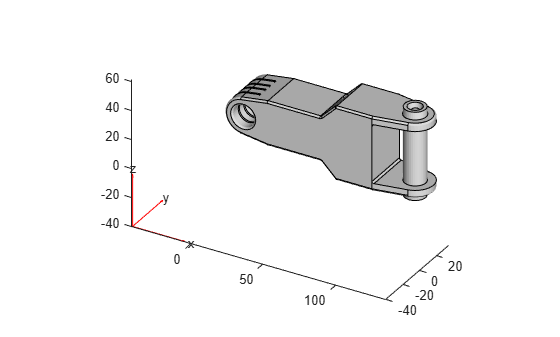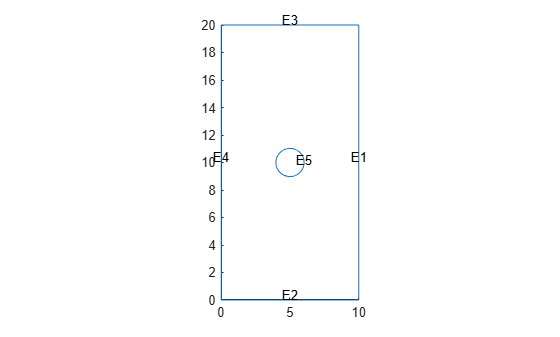# importGeometry

Import 2-D or 3-D geometry from STL data

## Syntax

``importGeometry(model,geometryfile)``
``gd = importGeometry(model,geometryfile)``

## Description

example

````importGeometry(model,geometryfile)` creates a geometry container from the specified STL geometry file, and includes the geometry in the `model` container.```
````gd = importGeometry(model,geometryfile)` also returns the geometry to the MATLAB® workspace.```

## Examples

collapse all

Import STL geometry into a PDE model.

Create a `PDEModel` container for a system of three equations.

`model = createpde(3);`

Import geometry into the container.

`importGeometry(model,'ForearmLink.stl');`

View the geometry with face labels.

`pdegplot(model,'FaceLabels','on')`Import a planar STL geometry into a PDE model. When importing a planar geometry, `importGeometry` converts it to a 2-D geometry by mapping it to the X-Y plane.

Create a `PDEModel` container.

`model = createpde;`

Import geometry into the container.

`importGeometry(model,'PlateHolePlanar.stl')`
```ans = DiscreteGeometry with properties: NumCells: 0 NumFaces: 1 NumEdges: 5 NumVertices: 5 ```

View the geometry with edge labels.

`pdegplot(model,'EdgeLabels','on')`## Input Arguments

collapse all

Model object, specified as a `PDEModel` object, `ThermalModel` object, or `StructuralModel` object.

Example: `model = createpde(1)`

Example: `thermalmodel = createpde('thermal','steadystate')`

Example: `structuralmodel = createpde('structural','static-solid')`

Path to STL file, specified as a character vector or a string scalar ending with the file extension `.stl` or `.STL`.

Example: `'../geometries/Carburetor.stl'`

Data Types: `char` | `string`

## Output Arguments

collapse all

Geometry description, returned as a `DiscreteGeometry` object. See DiscreteGeometry Properties for details.

## Limitations

• `importGeometry` does not allow you to import a multidomain 2-D or 3-D geometry where subdomains have any common points. If the subdomains of the geometry have common points, the toolbox still treats these subdomains as disconnected, without any common interface between them. Each subdomain has its own mesh.

Because of this limitation, you cannot import nested 3-D geometries directly. As a workaround, you can import a mesh and then create a multidomain geometry from the mesh by using the `geometryFromMesh` function.

## Tips

• STL format approximates the boundary of a CAD geometry by a collection of triangles, and `importGeometry` reconstructs the faces and edges from this data. Reconstruction from STL data is not precise and can result in a loss of edges and, therefore, the merging of adjacent faces. Typically, lost edges are the edges between two adjacent faces meeting at a small angle, or smooth edges bounding blend surfaces. Usually, the loss of such edges does not affect the analysis workflow.• Because STL geometries approximate the original CAD geometries, areas and volumes of STL and CAD geometries can differ.